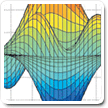Cody

# Problem 879. Perform Rubik's Cube Moves

A standard Rubik's Cube is shown in 3-D and also unfolded to identify the specific Tile-face numbering.

The faces are White-Up / Red-Left / Blue-Front / Orange-Right / Yellow-Down / Green-Back (ULFRDB)

Moves are denoted as F for clockwise rotation of the Front face. F' is CCW and F2 is F twice. The F move would map 19 to 25,..., 7 goes to 18,..., and 23 is unchanged.

The moves X( R ), Y(U), and Z(F) rotate the entire cube with its associated face. X would map 23 to 14. (19:27) moves to (10:18). Only contents of 5 and 50 are unchanged There are also X' and X2 moves.```Input: (mov,rubik)
mov: is an integer 1:27 representing the moves in order: UFDLBRU'F'D'L'B'R'U2F2D2L2B2R2XYZX'Y'Z'X2Y2Z2
rubik: row vector of size 54
(A normal cube would have values 0:5 in locations 1:54,
The test input is generally numbered 1:54 to check that each tile is correctly remapped. A normal cube with 6 values may be used in testing)
```
```Output: rubik vector of the input cubes values remapped based upon mov
```

All 27 moves are enumerated in the test suite

Follow-up Challenges will be solving the cube at various move depths for time and actual face moves required.

Note: Images inserted/linked to my free google web page. Used a gif and a png.

### Solution Stats

77.78% Correct | 22.22% Incorrect
Last Solution submitted on Aug 21, 2018